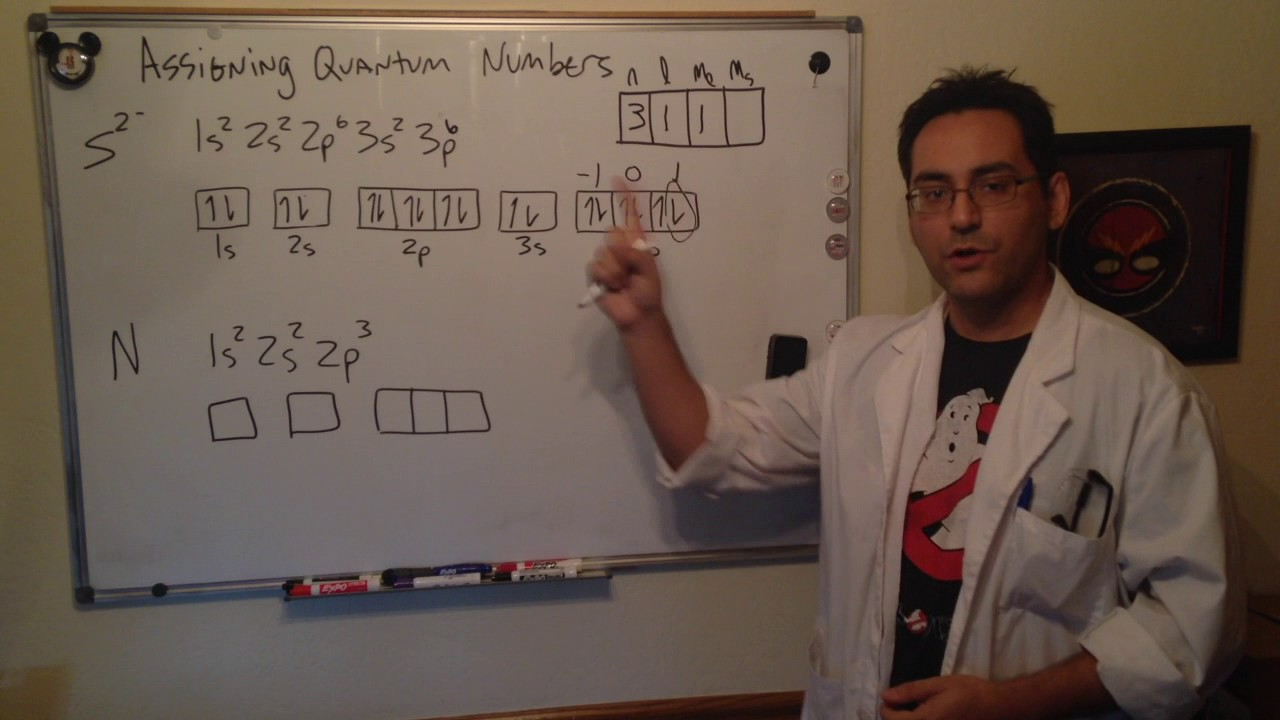# What are the Four Quantum Numbers that can be Assigned to Electrons?

The movement and the trajectory followed by an electron in an atom can be described with the help of quantum numbers. The four quantum numbers that can be employed for this purpose include:

• The principal quantum number, often denoted by the symbol ‘n’.
• The azimuthal quantum number (also known as the orbital angular momentum quantum number), often denoted by the symbol ‘l’.
• The magnetic quantum number, often denoted by the symbol ‘ml’.
• The electron spin quantum number, often denoted by the symbol ‘ms’.

## Principal Quantum Number

The principal electron shell of the atom is designated by the principal quantum number. The greater the value of the principal quantum number, the greater the distance between the electron and the nucleus. It is important to note that the principal quantum number can assume the value of any positive integer, which is greater than or equal to one (i.e. n = 1, 2, 3, 4, ….).

## Azimuthal Quantum Number or Orbital Angular Momentum Quantum Number

The shape of the orbital occupied by the electron in question is decided by the azimuthal quantum number. This quantum number is also known as the orbital angular momentum quantum number. The value of the orbital angular momentum quantum number is dependent on the value of the principal quantum number. Its values range from zero to n-1. Therefore, if the value of the principal quantum number is 2, the value of the azimuthal quantum number can be either zero or one.

## Magnetic Quantum Number

The magnetic quantum number decides the total number of orbitals that can exist in a subshell. This quantum number also decides the orientation of these orbitals. The values that can be assumed by the magnetic quantum number depend on the value of the azimuthal quantum number. These values range from -l to l. Therefore, if the value of the azimuthal quantum number is 1, the possible values of the magnetic quantum number are -1, 0, and 1.

## Electron Spin Quantum Number

This quantum number decides the spin of the electron. It can assume one of two different values, -½ and ½.